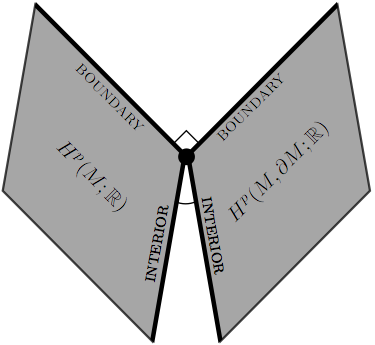# Clayton ShonkwilerIn my paper “Poincaré duality angles and the Dirichlet-to-Neumann operator”, which is a cleaned-up version of my dissertation, I introduce Poincaré duality angles, which measure the relative positions of concrete realizations of the absolute and relative cohomology groups of a Riemannian manifold with boundary inside the space of differential forms.

Both the $$p$$th absolute and the $$p$$th relative de Rham cohomology groups of a Riemannian manifold $$M$$ with boundary are represented by spaces of harmonic $$p$$-forms. As illustrated in the above image, the absolute and relative spaces both have an orthogonal decomposition into a subspaces corresponding to cohomology coming from the boundary and a subspace corresponding to cohomology coming from the interior of the manifold. The relative boundary subspace is orthogonal to the whole absolute subspace, and similarly the whole absolute boundary subspace is orthogonal to the whole relative subspace. However, principal angles between the interior subspaces are all strictly acute; these are the Poincaré duality angles.

It turns out that the Poincaré duality angles are closely related to the Dirichlet-to-Neumann operator for differential forms, which is a souped-up version of the voltage-to-current map at the boundary of a conducting medium.